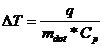NESS ENGINEERING
TECHNICAL DATA
WATER COOLING

Water cooling is often used in order to help dissipate or at least transfer large amounts of dissipated power (heat) which can be typical of many power electronics and repetitive pulsed power applications. The formulas on this page allow one to calculate the temperature rise for a given water cooling application where the power dissipation and flow rate are known. By knowing the density of water, one can determine the mass flow rate based on the volumetric flow rate and then solve for the temperature rise.DT is the temperature rise or Delta (Deg C) q is the heat load or dissipated power (W or J/sec)  mdot is the mass flow rate (gm/sec)  Cp is the specific heat of water (4.186 J/gm deg C)

The calculator below can be used to determine the temperature delta or rise for a given cooling water application (heat load or power dissipated and cooling water flow rate) using the first formula above. It will also determine the mass flow rate based on those parameters. Credit for the initial Javascript code used in the calculator is given to Ray Allen who has a number of similar useful calculators on his website, Pulsed Power Portal.

Calculate Cooling Water Temp Rise

Units

Inputs:

q (Power Dissipation or Heat Load)
f (Water Volumetric Flow Rate)

Outputs:

Delta T (Temperature Rise)

Mdot (Mass Flow Rate)

Output Format:

 Select Format: Scientific Engineering Fixed

Send consulting inquiries, comments, and suggestions to nessengr@san.rr.com

Ness Engineering, Inc.
P.O. Box 261501
San Diego, CA 92196
(858) 566-2372
(858) 240-2299 FAX

 © Richard M. Ness and Ness Engineering, Inc. Last Updated: